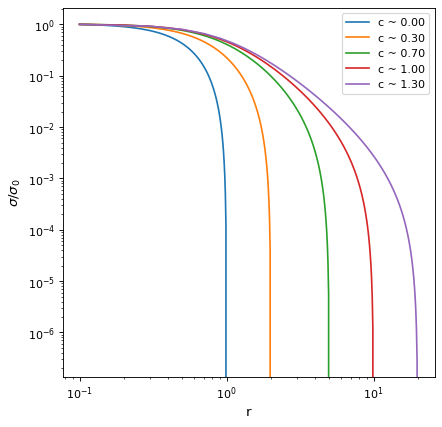# KingProjectedAnalytic1D¶

class astropy.modeling.functional_models.KingProjectedAnalytic1D(amplitude=1, r_core=1, r_tide=2, **kwargs)[源代码]

amplitude浮动

r_core浮动

r_tide浮动

fixed口述，可选

tied可选的

bounds可选的

eqcons可选列表

ineqcons可选列表

$f（x）=左（\frac{1}{\sqrt{（x^2+r}2）}}-$

1

import numpy as np
from astropy.modeling.models import KingProjectedAnalytic1D
import matplotlib.pyplot as plt

plt.figure()
rt_list = [1, 2, 5, 10, 20]
for rt in rt_list:
r = np.linspace(0.1, rt, 100)

mod = KingProjectedAnalytic1D(amplitude = 1, r_core = 1., r_tide = rt)
sig = mod(r)

plt.loglog(r, sig/sig, label='c ~ {:0.2f}'.format(mod.concentration))

plt.xlabel("r")
plt.ylabel(r"$\sigma/\sigma_0$")
plt.legend()
plt.show()


(png _, svgpdfking模型的浓度参数 此属性用于指示evaluate方法所需的单元或单元集，并返回将输入映射到单元（或 None 如果接受任何单位）。 描述此类型模型的参数的名称。

 evaluate \（x，振幅，r 核心，r u潮汐） 解析金模型函数。 fit_deriv \（x，振幅，r 核心，r u潮汐） 解析金模型函数导数。

amplitude = Parameter('amplitude', value=1.0, bounds=(1.1754943508222875e-38, None))
concentration

king模型的浓度参数

input_units
param_names = ('amplitude', 'r_core', 'r_tide')

r_core = Parameter('r_core', value=1.0, bounds=(1.1754943508222875e-38, None))
r_tide = Parameter('r_tide', value=2.0, bounds=(1.1754943508222875e-38, None))

static evaluate(x, amplitude, r_core, r_tide)[源代码]

static fit_deriv(x, amplitude, r_core, r_tide)[源代码]